Work Power Energy Exam2 and Problem Solutions

Work Power Energy Exam2 and  Problem Solutions

1. Applied force vs. position graph of an object is given below. Find the kinetic energy gained by the object at distance 12m.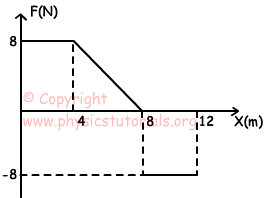By using work and energy theorem we say that; area under the graph gives us work done by the force.

ΔEK=W=area under the graph=(8+4)/2.8-8(12-8)

ΔEK=12.4-8.4=16 joule

2. Box having mass 3kg thrown with an initial velocity 10 m/s on an inclined plane. If the box passes from the point B with 4m/s velocity, find the work done by friction force.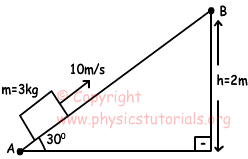We use conservation of energy theorem.

EA=EB+Wfriction

Wfriction=1/2.m.V2-(mgh+1/2mVL2)

Wfriction=1/2.3.102-(3.10.2+1/2.3.42)

Wfriction=66 joule

3. Three different forces are applied to a box in different intervals. Graph, given below, shows kinetic energy gained by the box in three intervals. Find the relation between applied forces.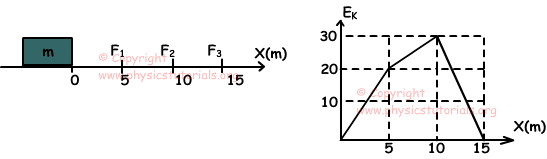Slope of the EK vs. position graph gives applied force

I. interval: F1=(20-0)/(5-0)=4N

II. interval: F2=(30-20)/(10-5)=2N

III. interval: F3= (0-30)/(15-10)=-6N

FIII>FI>FII

4. A stationary object at t=0, has an acceleration vs. time graph given below. If object has kinetic energy E at t=t, find the kinetic energy of the object at t=2t in terms of E.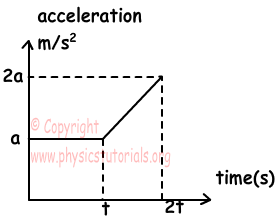Object has velocity at t=t;

V1=at

Object has velocity at t=2t;

V2=at+((2a+a)/2).t=at+3/2.at=5/2.at

V2=5/2.V1

E2/E=(1/2.m.V22)/(1/2.m.V12)=(5/2.V1)2/V12

E2/E=25/4

E2=25E/4

5. An object does free fall. Picture given below shows this motion. Find the ratio of kinetic energy at point C to total mechanical energy of the object.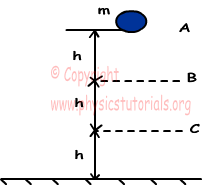Object lost 2mgh potential energy from point A to C. According to conservation of energy theorem, this lost potential energy converted to the kinetic energy. Thus; we can say that kinetic energy of the object at point C is;

EK=2mgh

Total mechanical energy;

Etotal=3mgh

EK/Etotal=2mgh/3mgh

Author: